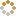﻿ Ningbo University of Technology Online Judge ::  Sliding Window
• ###  Sliding Window

• 时间限制: 5000 ms　内存限制: 131072 K
• 问题描述
• An array of size n ≤ 10^6 is given to you. There is a sliding window of size k which is moving from the very left of the array to the very right. You can only see the k numbers in the window. Each time the sliding window moves rightwards by one position. Following is an example:
The array is [1 3 -1 -3 5 3 6 7], and k is 3.

Window position Minimum value Maximum value
[1 3 -1] -3 5 3 6 7 -1 3
1 [3 -1 -3] 5 3 6 7 -3 3
1 3 [-1 -3 5] 3 6 7 -3 5
1 3 -1 [-3 5 3] 6 7 -3 5
1 3 -1 -3 [5 3 6] 7 3 6
1 3 -1 -3 5 [3 6 7] 3 7

Your task is to determine the maximum and minimum values in the sliding window at each position.

• 输入
• There are N cases(1 <= N < 10).
For each case, it consists of two lines. The first line contains two integers n and k which are the lengths of the array and the sliding window. There are n integers in the second line.
Input until EOF.
• 输出
• There are two lines in the output for each case. The first line gives the minimum values in the window at each position, from left to right, respectively. The second line gives the maximum values.

Be careful that in the end of each line, there's no space " ".
• 样例输入
• ```8 3
1 3 -1 -3 5 3 6 7
```
• 样例输出
• ```-1 -3 -3 -3 3 3
3 3 5 5 6 7
```
• 提示
• `无`
• 来源
• ```POJ Monthly -- 2006.04.28, Ikki
```
• 操作
﻿